# 12-129. The car starts from rest at s =0 and increases itsspeed at a, =magnitude of acceleration becomes 20 m/s?. At whatposition s does this occur?4 m/s2. Determine the time when the%3DDetegrssd nis8lo bosnogorbon i natwp = 40 m%3D

Question
121 views

Specifically a dynamics problem. Question and diagram in pic below.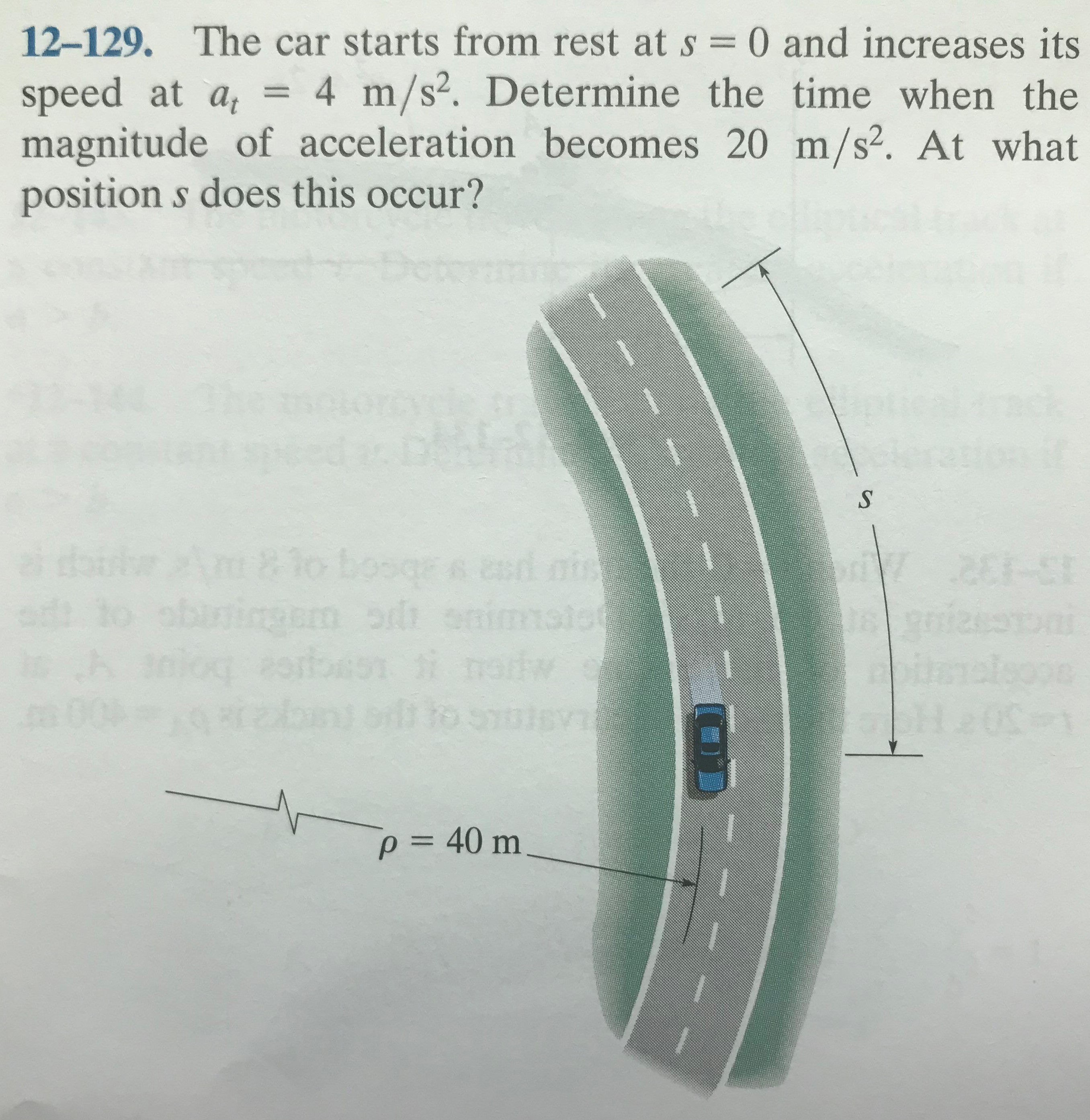help_outlineImage Transcriptionclose12-129. The car starts from rest at s =0 and increases its speed at a, = magnitude of acceleration becomes 20 m/s?. At what position s does this occur? 4 m/s2. Determine the time when the %3D Dete grssd nis 8lo bos nogorbon i natw p = 40 m %3D fullscreen
check_circle

Step 1

The car starts from rest at s = 0 and increases its speed at the tangential acceleration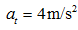The final acceleration is 20 m/s2.

Now, from the equation of motion,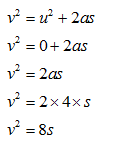Step 2

The value of normal acceleration is given by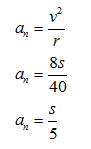Step 3

Now, the acceleration is given as 20 m/s2, with the help of this the value of distance s can be calculated.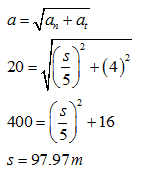...

### Want to see the full answer?

See Solution

#### Want to see this answer and more?

Solutions are written by subject experts who are available 24/7. Questions are typically answered within 1 hour.*

See Solution
*Response times may vary by subject and question.
Tagged in

### Mechanical Engineering